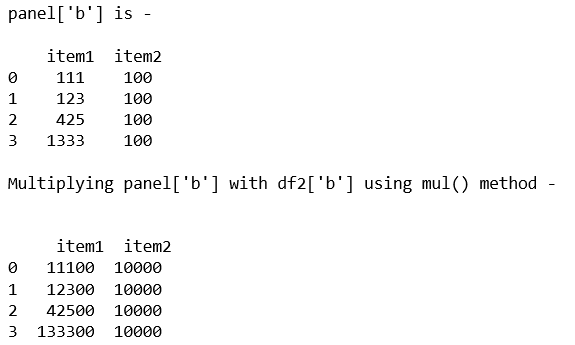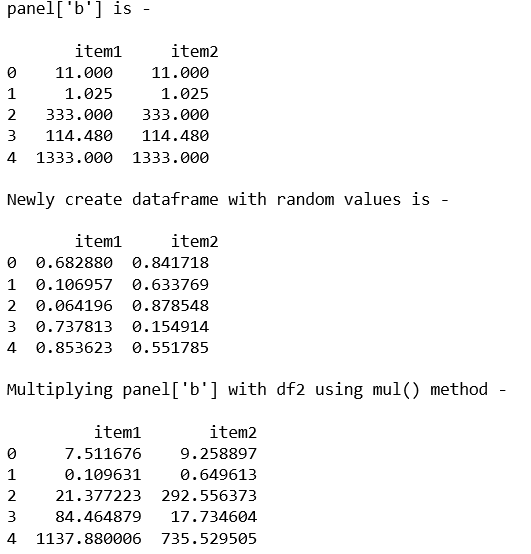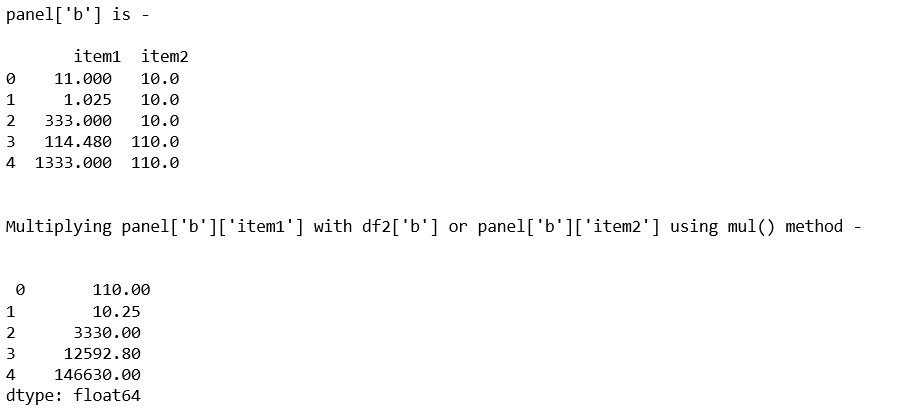Related Articles

# Python | Pandas Panel.mul()

• Last Updated : 28 Jan, 2019

In Pandas, Panel is a very important container for three-dimensional data. The names for the 3 axes are intended to give some semantic meaning to describing operations involving panel data and, in particular, econometric analysis of panel data.

In Pandas `Panel.mul()` function is used to get the multiplication of series and dataframe/Panel.

Attention geek! Strengthen your foundations with the Python Programming Foundation Course and learn the basics.

To begin with, your interview preparations Enhance your Data Structures concepts with the Python DS Course. And to begin with your Machine Learning Journey, join the Machine Learning - Basic Level Course

Syntax: Panel.mul(other, axis=0)

Parameters:
other : DataFrame or Panel
axis : Axis to broadcast over

Returns: Panel

Code #1:

 `# importing pandas module ``import` `pandas as pd ``import` `numpy as np `` ` `df1 ``=` `pd.DataFrame({``'a'``: [``'Geeks'``, ``'For'``, ``'geeks'``, ``'real'``], ``                    ``'b'``: [``111``, ``123``, ``425``, ``1333``]}) `` ` `df2 ``=` `pd.DataFrame({``'a'``: [``'I'``, ``'am'``, ``'dataframe'``, ``'two'``], ``                    ``'b'``: [``100``, ``100``, ``100``, ``100``]}) ``                     ` `data ``=` `{``'item1'``:df1, ``'item2'``:df2}`` ` `# creating Panel ``panel ``=` `pd.Panel.from_dict(data, orient ``=``'minor'``) ``print``(``"panel['b'] is - \n\n"``, panel[``'b'``]) `` ` `print``(``"\nMultiplying panel['b'] with df2['b'] using mul() method - \n"``) ``print``(``"\n"``, panel[``'b'``].mul(df2[``'b'``], axis ``=` `0``)) `

Output:Code #2:

 `# importing pandas module ``import` `pandas as pd ``import` `numpy as np `` ` `df1 ``=` `pd.DataFrame({``'a'``: [``'Geeks'``, ``'For'``, ``'geeks'``, ``'for'``, ``'real'``], ``                    ``'b'``: [``11``, ``1.025``, ``333``, ``114.48``, ``1333``]}) `` ` `data ``=` `{``'item1'``:df1, ``'item2'``:df1} `` ` `# creating Panel ``panel ``=` `pd.Panel.from_dict(data, orient ``=``'minor'``) ``print``(``"panel['b'] is - \n\n"``, panel[``'b'``], ``'\n'``) `` ` `# Create a 5 * 5 dataframe ``df2 ``=` `pd.DataFrame(np.random.rand(``5``, ``2``), columns ``=``[``'item1'``, ``'item2'``]) ``print``(``"Newly create dataframe with random values is - \n\n"``, df2)`` ` `print``(``"\nMultiplying panel['b'] with df2 using mul() method - \n"``) ``print``(panel[``'b'``].mul(df2, axis ``=` `0``)) `

Output:Code #3:

 `# importing pandas module ``import` `pandas as pd ``import` `numpy as np `` ` `df1 ``=` `pd.DataFrame({``'a'``: [``'Geeks'``, ``'For'``, ``'geeks'``, ``'for'``, ``'real'``], ``                    ``'b'``: [``11``, ``1.025``, ``333``, ``114.48``, ``1333``]}) ``                     ` `df2 ``=` `pd.DataFrame({``'a'``: [``'I'``, ``'am'``, ``'DataFrame'``, ``'number'``, ``'two'``], ``                    ``'b'``: [``10``, ``10``, ``10``, ``110``, ``110``]})                     ``                     ` `data ``=` `{``'item1'``:df1, ``'item2'``:df2} `` ` `# creating Panel ``panel ``=` `pd.Panel.from_dict(data, orient ``=``'minor'``) `` ` `print``(``"panel['b'] is - \n\n"``, panel[``'b'``], ``'\n'``) `` ` `print``(``"\nMultiplying panel['b']['item1'] with df2['b'] or panel['b']['item2'] using mul() method - \n"``) ``print``(``"\n"``, panel[``'b'``][``'item1'``].mul(df2[``'b'``], axis ``=` `0``)) `

Output:My Personal Notes arrow_drop_up